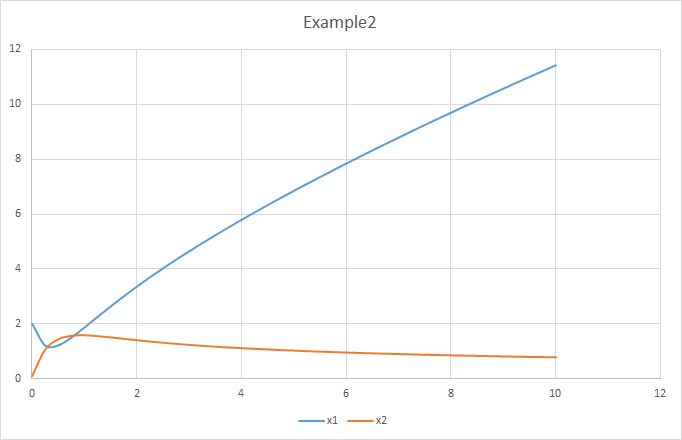x = a * x1 ^ a1 * x2 ^ a2 * ... - b * x1 ^ b1 * x2 ^ b2 * ...

• 如果对应的幂指数项为零，则对应的部分为1，将不会影响乘积结果，即对应的符号与x的变化无关
• 如果对应的幂指数项大于零，则表示催化效应，因为对应的幂的结果总是大于1的
• 反之如果对应的幂指数项小于零，则表示抑制效应。一个符号越大，其负的幂指数结果会越接近于零，整个乘积项将会接近于零，故表现为抑制效应。

• x4为净流入，其变化量为一个常数值
• x3同样在系统内为净流入，所以其变化量也是一个常数值
• 对于x2而言，其从x4转换而来，并且转换为x1，所以a部分为x4相关，本部分为其自身降解部分。在x4转换为x2的过程，受x1催化，x3抑制，所以x1的幂指数大于零，x3的幂指数小于零
• 对于x1而言，其来自于x2的转化，并且不受其他因素影响，所以a部分只与x2相关。在消耗部分，因为受到x3的抑制，所以b部分的表达式会与x3相关，并且x3的幂指数小于零

[
x1 -> 2 * x2 - 1.2 * (x1 ^ 0.5) * (x3 ^ (-1)),
x2 -> 2 * (x1 ^ 0.1) * (x3 ^ (-1)) * (x4 ^ 0.5) - (2 * x2),
x3 -> 0.5,
x4 -> 1
]
|> deSolve(
y0 = list(
x1 = 2,
x2 = 0.1,
x3 = 0.5,
x4 = 1
),
a          = 0,
b          = 10,
resolution = 20000
)
|> as.data.frame
|> write.csv(file = \${dirname(@script)}/example2.csv)
;X1变量首先会因为X3浓度较低，抑制力作用较低而在初期有一个较大的消耗量。在曲线上首先展现出一个被消耗的趋势，之后由于X3浓度不断累积，X1的消耗反应接近停止，X1表现出在后期不断地累积；
X2变量而言，由于其通过X4转化的过程受X3抑制，因为X3后期不断累积，所以X4转化为X2的过程在不断的受抑制，X2的转化效率低于其转化为X1的时候，X2的浓度将会不断降低

## GCModeller之中内置的PLAS计算引擎

• snapshot 函数用来打开一个用来进行本地数据存储的文件设备，用来保存模拟器输出的每一帧符号数据，可以从下面的代码看到，这个函数接受一个符号列表用来选择性的输出结果数据
• kernel 函数用来创建一个基于S-system算法的生物化学系统动力学模拟器对象
• environment 函数就是用来配置kernel中的一些符号常量等信息
• s.system 函数就是用来向模拟器核心加载我们所定义的S-system系统方程
• run 最后run函数就是执行整个模拟器的运行

### PLAS模拟器脚本示意

imports "S.system" from "simulators";

# set y0 of the ODEs system
const symbols = list(
x1 = -100,
x2 = -100000,
x3 = 0,
x4 = 10
);

using data.driver as snapshot("./atkinson.csv", symbols = names(symbols)) {
data.driver
|> kernel(S.script("Atkinson system"))
|> environment(
beta1    = 30,
beta3    = 30,
beta4    = 1,
lamda1   = 2,
lamda3   = 2,
alpha1   = 20,
alpha2   = 20,
alpha3   = 1,
a        = 1,
n1       = 4,
n2       = 5,
n3       = 1,
is.const = TRUE
)
|> environment(symbols)
|> s.system([
x1 -> beta1 * (lamda1 * (1 + alpha1 * (x4 ^ n1) / (1 + x4 ^ n1)) - x1),
x2 -> x1 - x2,
x3 -> beta3 * (lamda3 * (1 + alpha2 * ((x4 / a) ^ n2) / (1 + (x4 / a) ^ n2)) * (1 / (1 + x2 ^ n3)) - x3),
x4 -> beta4 * (x3 - x4)
])
|> run(ticks = 4.5, resolution = 0.1)
;
}Latest posts by 谢桂纲 (see all)

### Order by Date Name Attachments

1.2.3.4.5.